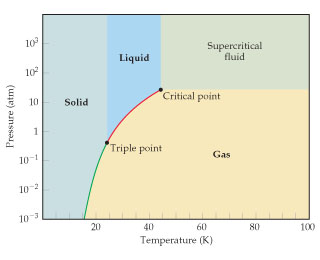# Problem: Use the phase diagram for neon to answer the following questions:Below what pressure value will solid neon sublime?

🤓 Based on our data, we think this question is relevant for Professor Dixon's class at UCF.

###### FREE Expert Solution
• Sublimation is the phase transition from solid to gas. Use the phase diagram to determine the pressure where solid turns directly to gas
• Analyzing the phase diagram, the green line appears to be the boundary of solid and gas phase which means that at any point of that particular line, solid and gas exists at the same time###### Problem Details

Use the phase diagram for neon to answer the following questions:Below what pressure value will solid neon sublime?